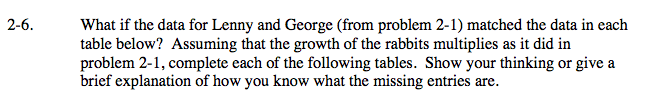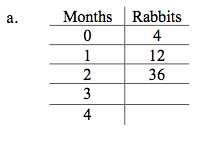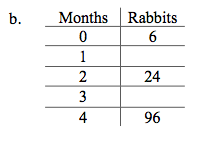Home > A2C > Chapter 2 > Lesson 2.1.1 > Problem2-6

2-6.
1. What if the data for Lenny and George (from problem 2-1) matched the data in each table below? Assuming that the growth of the rabbits multiplies as it did in problem 2-1, complete each of the following tables. Show your thinking or give a brief explanation of how you know what the missing entries are. Homework Help ✎

1.  Months Rabbits 0 4 1 12 2 36 3 4
2.  Months Rabbits 0 6 1 2 24 3 4 96What could be the multiplier? Check it with given values.

Use the multiplier to determine the missing values.

3 Months = 108 Rabbits;
4 Months = 324 Rabbits.What could be the multiplier? Check it with given values.

Use the multiplier to determine the missing values.

1 Month = 12 Rabbits
3 Months = 48 Rabbits